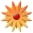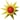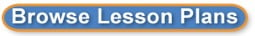# Probability- How Likely Is It?

Page Views: 3009

Email This Lesson Plan to Me
Overall Rating:(5.0 stars, 1 ratings)

 Keywords: Probability, Math, Experiment, Manipulatives, Glogster.com Subject(s): Math Grades 5 through 6 NETS-S Standard: Creativity and InnovationCommunication and CollaborationCritical Thinking, Problem Solving, and Decision MakingTechnology Operations and ConceptsView Full Text of Standards School: Port Clinton Middle School, Port Clinton, OH Planned By: Sarah Piskos Original Author: Sarah Piskos, Port Clinton
Throughout our probability unit, we have discussed what it means to determine how likely an event is, based on given parameters. We talked about experimental and theoretical probabilities, and we even acted out some situations to study probabilities, patterns and outcomes.

To wrap up this unit, students will be completing a project either individually, or with a partner. This is different from most projects, though, in that STUDENTS get to pick what the topic is! Based on what we have learned so far, what interests students, and interactive tools available online, students will be choosing a situation, its parameters, and a desired outcome, and will ultimately study the probability of that outcome by utilizing some cutting edge technology.

Whatever topic students choose to study, they must include ALL of the following:

(1) An explanation of their topic, its parameters, and the desired outcome.

(2) Students must explore interactive sites online to find a tool to simulate their situation.
o Example: If I am studying coin tosses, I would need to find a simulation of a coin toss online.
o See below for suggested websites to explore.

(3) Students should simulate their experiment a MINIMUM of 30 times online, and record the data in a spreadsheet using Excel.

(4) After collecting their data and documenting it in a spreadsheet, students should use the formula tools in Excel to calculate the totals for each possible outcome, AND the averages of each possible outcome.

(5) After completing the calculations described above, students will use Excel to create a bar chart AND a circle graph to display their conclusions.

(6) To share their findings with the class, students need to create a Glogster poster online. This poster should include
o Their topic and parameters.
o The 2 charts from Excel.
o Links to the interactive sites used online.

(7) When finished with the poster, students need to do 2 things:
1. Email the teacher a link to the poster online.
2. Post a link to the poster on a class wiki.

(8) Finally, when all parts of the project are completed, students will share with the class their topic, how they simulated the experiment using cutting edge technology, and conclusions found. If working with a partner, BOTH students must share some information!

***If needed, students should be given short tutorials about how to use Excel functions, Excel charts, and the Glogster website.

Students should have class time to work on this project, but some work might be homework. _________________________________________________________________________
InteractiveProbability Websites

Below are numerous interactive sites that would be appropriate for this lesson. They are categorized by URL address, and I have included short descriptions of the manipulatives on each site that might be useful.

Students are NOT limited to these websites, but they are a start. If students find a website not listed below, please check with the teacher for approval.

http://www.shodor.org/interactivate/activities/
Scroll
down to where it says Probability (24)

Run a simulation of how a fire might spread through trees, learning about probability and chaos. Track the results of multiple burns and use the data to draw conclusions.
Create a spinner with variable sized sectors to look at experimental and theoretical probabilities. Parameters: Sizes of sectors, number of sectors, number of trials.
Choose one of N doors to determine the odds of winning the grand prize behind that door, similar to the TV program "Let's Make a Deal." Parameters: Number of doors, number of trials, staying or switching between the two remaining doors.
4. Coin Toss
Simulations of a coin toss allowing the user to input the number of flips. Toss results can be viewed as a list of individual outcomes, ratios, or in a table.
5. Crazy Choices Game
Compare theoretical and experimental probabilities, using dice, cards, spinners, or coin tosses. Three different probabilities can be compared at once. Parameters: Type of probabilities, number of trials.
6. Dice Table
Experiment with the outcome distribution for a roll of two dice by simulating a dice throwing game. Parameters: Which player wins with which total rolled.
7. Experimental Probability
Experiment with probability using a fixed size section spinner, a variable section spinner, two regular 6-sided dice or customized dice.
8. Marbles
Learn about sampling, with and without replacement, by randomly drawing marbles from a bag. Parameters: Number and color of marbles in the bag, replacement rule.
Models how a population of susceptible, infected, and recovered people is affected by a disease.

National
Library of Virtual Manipulatives
Scroll down to Data Analysis and Probability (Grades 6-8)

1. Bar Chart  Create a bar chart showing quantities or percentages by labeling columns and clicking on values.
2. Box Model  Randomly selects and displays the removal of various objects from a box.
3. Coin Tossing  Explore probability concepts by simulating repeated coin tosses.
4. Hamlet Happens  Verify that rare events happen by drawing letters from a box.
5. Spinners  Work with spinners to learn about numbers and probabilities.
6. Stick or Switch  Investigate probabilities of sticking with a decision, or switching your mind.

http://www.teachers.cr.k12.de.us/~galgano/cmp/six.htm
Scroll
down to How Likely Is It?

1. Coin Tossing - Simulates repeated coin tosses.
2. Pick-a Block - Simulates picking colored blocks.
3. Spinner - Create a spinner with 1-12 sectors to look at probabilities.
4. Random Drawing Tool  Explore theoretical and experimental probability.
5. Experimental Probability - Experiment with experimental probability.
6. Racing Game (One Die) - 2 players roll a die, and move toward the finish.
7. Racing Game (2 Dice)  N players roll 2 dice, and move toward the finish.
8. Crazy Choices Game - 3 players play games using dice, cards, spinners or coin tosses, to compare theoretical & experimental probabilities.
9. Hamlet Happens  Study rare events by drawing letters from a box.
10. Socks in the Dryer  Study a given probability problem: 4 pairs of socks of 4 different colors go in the dryer, and randomly, 4 socks are eaten each time.

http://www.sdb.k12.wi.us/cmp/figure-this-gr-6.html#prime
Scroll
down to How Likely Is It?

1. Figure This - Wheel of Fortune
2. Next Level of Challenge  Simulates probability situations
3. Games & Activities - Remove one (AKA Roller Derby)

***You might find this website useful if you need help brainstorming a topic, or if you want more information about probability content.

1. Probability Video- Bill Nye, the Science Guy, explains Probability
2. Probability Problems
 Making Tree Diagrams for Possible Outcomes
 Independent & Dependent Events
3. Probability Worksheets
 Probability Problems (I)
 Complementary Probability
 Probability Problems (II)
4. Games
 Probability Games
 Comments Depending upon student resources at home (i.e. computers, internet, spreadsheet software, etc.), this might need to be an activity completed entirely in school. Cross-Curriculum Ideas This math experiment connects closely with the scientific method. Students are formulating a question, predicting/considering outcomes, experimenting, and drawing conclusions. Follow-Up To continue the topic of probability, students can contribute questions/comments regarding their probability experiment to a class wiki that classmates can respond to, and explore. Links: Link to glogster.com Materials: Mobile Labs, Projectors, Short Throw Projectors, Projector Screens, Calculators, Middle School, Hard Drives, Printers, Computer Accessories, Power, Keyboards, Mice, Ports and Hubs, Math, Middle, Office Suite, Word Processor, Spreadsheet, Database, Keyboarding, Internet Services